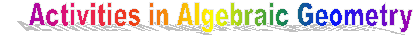M
C
G
I
L
L

M
A
T
H
E
M
A
T
I
C
S

M
C
G
I
L
L

M
A
T
H
E
M
A
T
I
C
SCourses (current and recent years):

COMING YEAR (2001-02):

Introduction to Algebraic Geometry. Lecturer: E. Goren.  This is an introductory course in Algebraic Geometry. It develops from scratch the theory of algebraic varieties over algebraically closed fields, including morphisms, sheaves and cohomology. The course presupposes basic commutative algebra. The topics to be studied are: affine and projective algebraic
varieties; regular functions and morphisms; singularities, normalization and blow-up; sheaves, Cech cohomology and the
Riemann-Roch theorem for curves. The examples of curves, and Grassmannian manifolds are studied in detail.

Vecotr bundles on Curves. Lecturer: E. Goren.  This is a special graduate course given within the Theme Year on Groups and Geometry taking place at the CRM during 2001-02. The course presupposes basic algebraic geometry at the level of the course \emph{Introduction to Algebraic Geometry} given in the first term. It develops the theory of curves and vector bundles of curves and their moduli. The topics to be studied are: curves, the Riemann-Roch theorem and the Hurwitz genus formula; vector bundles on curves and their invariants, monodromy, flat vector bundles and Weil's theorem; semi-stability; the Hilbert scheme; geometric invariant theory; construction of moduli spaces.

1997-1998:

Commutative algebra and algebraic geometry (Algebre 1). UdM. MAT 6608. Lecturer: B. Broer. Automn 97.

Topics in Algebra III: Approaches to the Jacobian Problem. McGill. 189-722A. Lecturer: A. Sathaye.

1998-1999:

Algebraic surfaces. McGill University. 189-725A. Lecturer: P. Russell. (A semester course leading to the study of systems of curves on an algebraic surface and the notion of Kodaira dimension for compact and non-compact surfaces).

Algebraic Curves. McGill. 189-612B. Lecturer:J. Hurtubise.

1999-2000:

Topics in Geometry and Topology . McGill University. 189-706A/707B. Lecturer: E. Goren. (A year long course in algebraic geometry, from classical theory to schemes. The course is aimed at the graduate, or advanced undergraduate level.  Pre-requisites are some basic algebra and topology. For more details go here. )

2000-2001:
Introduction to Riemannian symmetric spaces. (Topics in Geometry and Topology I). McGill 189-706A, MW 10:30 - 12:00, Room 1214. Lecturer: F. Andreatta.  The course is aimed at the beginning graduate student level and higher, and is open to advanced undergraduate students. After introduction of the necessary background in differential geometry and Lie theory, the focus would be the notion of symmetric spaces, their properties, their relation and classification in terms of Lie groups due to Cartan. Applications and connections to number theory and arithmetic algebrai geometry (e.g. classification of variations of Hodge structures, Shimura varieties) would be given in detail depending on time.

Elliptic Curves (Topics in Algebra). Concordia MAST 699I/4. Lecturer: Chantal David. This course will cover the basic theory of elliptic curves and algebraic curves. The recommended textbook is: The arithmentic of elliptic curvesby J. Silverman. The prerequisites for this course are part of the standard background in abstract algebra (groups, rings, field extensions etc). A
first course in algebraic number theory is also recommended. The course is open to all graduate students, either at the master or the Ph.D. level.

The graduate students seminar is devoted this year (1999-2000) to Faltings' Theorems. The proofs involve sophisticated tools from arithmetic algebraic geometry and algebraic number theory.

Seminars

See also the activities of